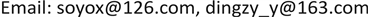STM32，酒精传感器，SIM800C，数学模型，数字滤波, STM32 Alcohol Sensor SIM800C Mathematical Model Digital Filtering

1淮海工学院电子工程学院，江苏 连云港

2江苏苏云医疗器材有限公司，江苏 连云港1. 引言

2. 系统结构设计

3. 硬件电路设计

3.1. 酒精检测电路设计

3.2. 温湿度检测电路设计

DHT11有专用的数字模块采集和温湿度检测传感技术。DHT11数字温湿度检测器件可以直接和STM32连接。DHT11的供电电压为3~5.5 V。当DHT11上电以后，要等待一秒，等待期间不用发送命令。其数据包由5个Byte (40 Bit)组成。

3.3. 超声波测距检测模块设计

3.4. 电源电路设计

1) 5 V转3.3 V最常用且简单的方法就是电阻分压法。5 V电平经一个1.6 k电阻和一个3.3 k电阻分压，此时分出的电压就是3.3 V。

2) 另一种方法就是使用电平转换芯片。因此在本系统中，我们采用的是AMS1117作为电平转换芯片。电容是为了滤掉纹波。

3.5. 显示电路设计

3.6. 通信电路设计

4. 酒精浓度检测算法设计4.1. 酒精浓度扩散的数学模型4.1.1. 模型的假设

1) 酒精浓度的传播可以简单地看做是空中某一点向四周等强度的瞬时释放，酒精在无穷空间扩散，不计风力和大地的影响。

2) 酒精浓度的扩散服从扩散定律。即单位时间通过单位法向面积的流量与它的浓度梯度成正比。

4.2.2. 模型的建立

q = − k × g r a d C (1)

Q 1 = ∫ t t + Δ t ∬ S q ⋅ n d σ d t (2)

Q 2 = ∭ V [ C ( x , y , z , t ) − C ( x , y , z , t + Δ t ) ] d V (3)

Q 1 = Q 2 (4)

∬ S q ⋅ n d σ = ∭ V d i v q d v (5)

d i v 是散度记号。根据(1)-(5)式，由积分中值定律得

∂ C ∂ t ≈ k d i v ( g r a d C ) = k ( ∂ 2 C ∂ x 2 + ∂ 2 C ∂ y 2 + ∂ 2 C ∂ z 2 ) ,     t > 0 , − ∞ < x , y , z < ∞ (6)

C ( x , y , z , 0 ) = Q δ ( x , y , z ) (7)

Q 表示呼气时释放的酒精总浓度， δ ( x , y , z ) 是单位发光强度的点源函数。

C ( x , y , z , t ) = Q ( 4 π k t ) 3 2 e − x 2 + y 2 + z 2 4 k t (8)

4.2. 数据处理4.2.1. 限速滤波法

4.2.2. 滑动平均滤波算法

4.3. 呼气中乙醇含量和血液中乙醇含量的关系

5. 系统调试

6. 总结